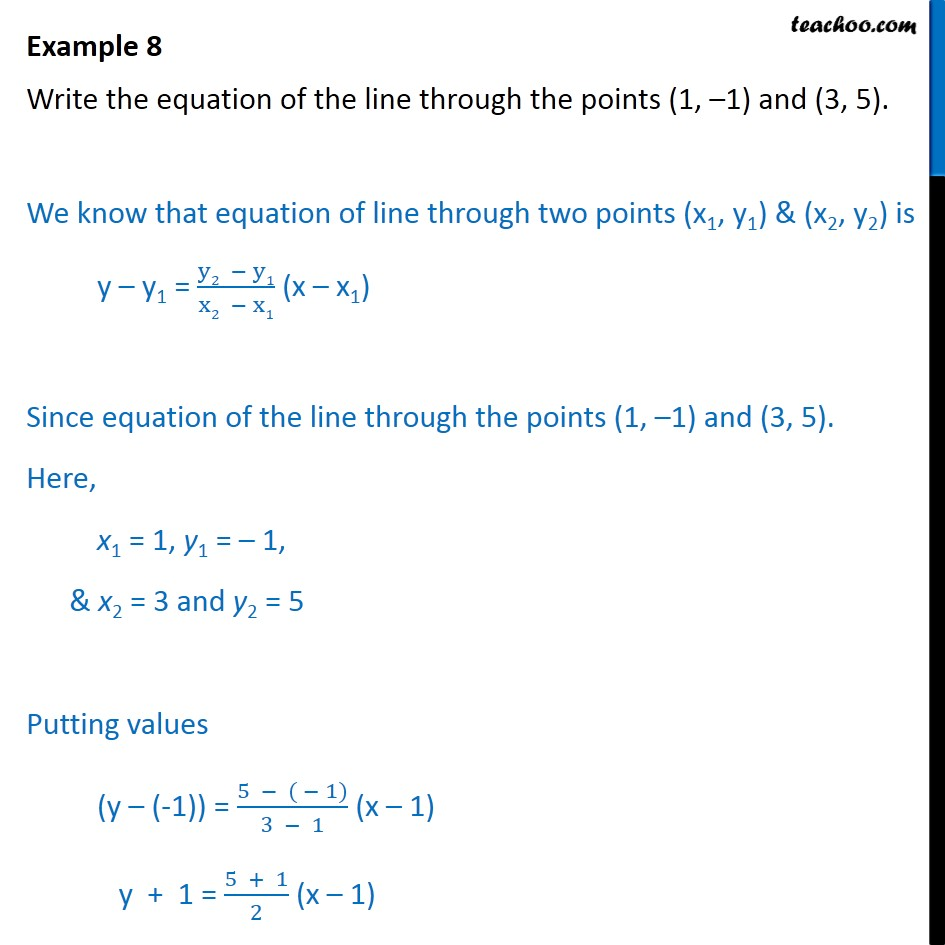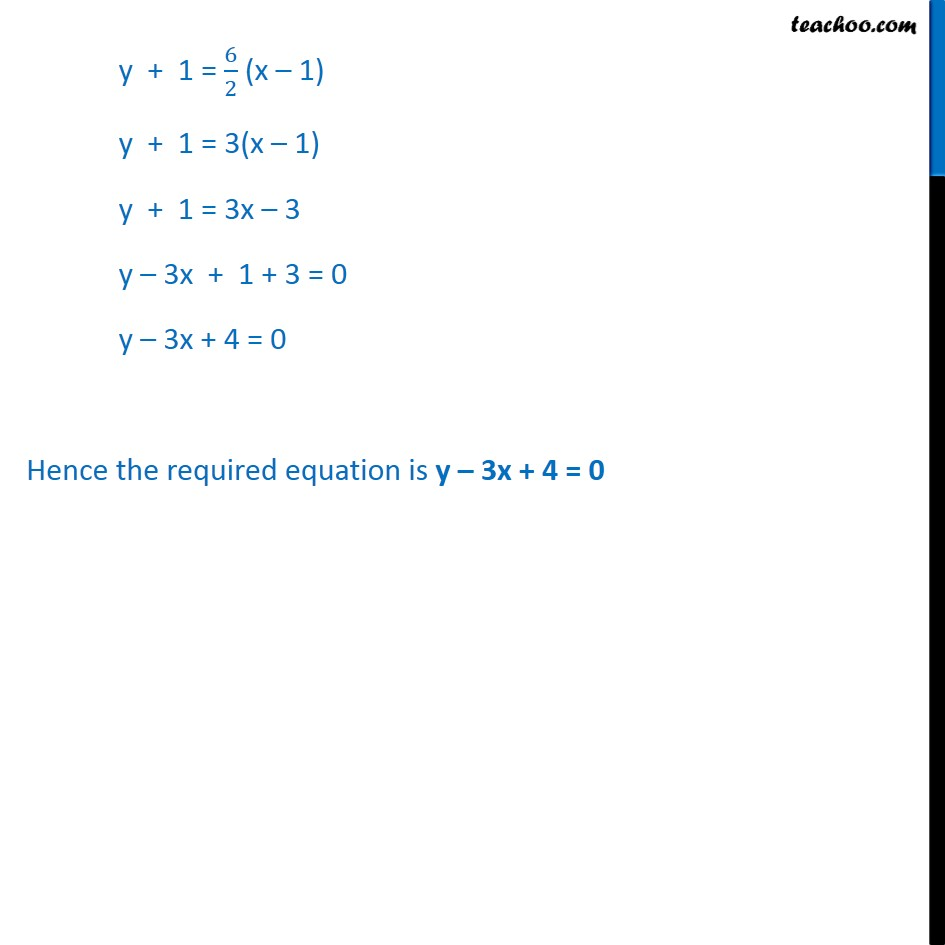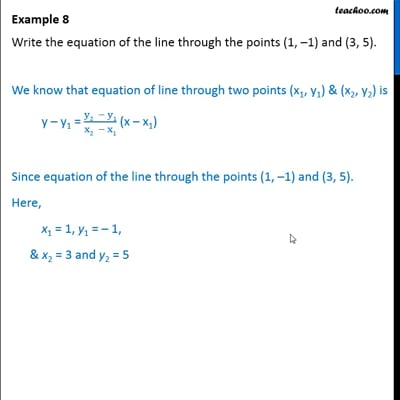Examples

Chapter 9 Class 11 Straight Lines
Serial order wiseThis video is only available for Teachoo black users

Learn in your speed, with individual attention - Teachoo Maths 1-on-1 Class

### Transcript

Example 6 Write the equation of the line through the points (1, 1) and (3, 5). We know that equation of line through two points (x1, y1) & (x2, y2) is y y1 = (y2 y1)/(x2 x1) (x x1) Since equation of the line through the points (1, 1) and (3, 5). Here, x1 = 1, y1 = 1, & x2 = 3 and y2 = 5 Putting values (y (-1)) = (5 ( 1))/(3 1) (x 1) y + 1 = (5 + 1)/2 (x 1) y + 1 = 6/2 (x 1) y + 1 = 3(x 1) y + 1 = 3x 3 y 3x + 1 3 = 0 y 3x 2 = 0 Hence the required equation is y 3x 2 = 0